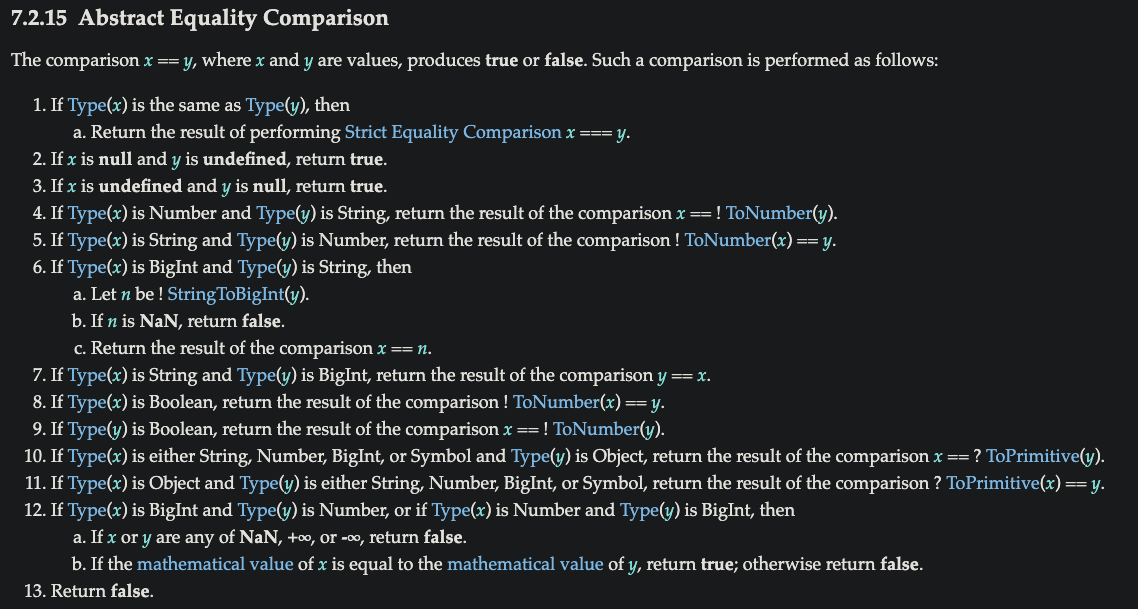#  強制轉型 - 寬鬆相等 ( == ) vs. 嚴格相等 ( === )

keywords:`loose equals`,`strict equals`

## 寬鬆相等（ loose equals ）vs. 嚴格相等（ strict equals ）

• 一般的描述是：『 == 檢查值 ( values ) 的相等性，=== 檢查值 ( values ) 及型別 ( types ) 的相等性 』 但這是錯的！！
• 正確的描述應該是：『 == 允許相等性比較中的強制轉型，而 === 不允許強制轉型 』

### 相等性的效能

• 一般描述中 === 所做的工作看似比 == 還要多，因為它也得檢查型別，但在正確的描述中會發現 == 才是作了比較多工作的一方，因為型別不同的話還得依循強制轉型的步驟
• 如果想要強制轉型就使用 == 寬鬆相等性，不想要強制轉型就使用 === 嚴格相等性。因為這兩者都會檢查它們的運算元的型別，差別就在於如果型別不符合，要如何反應。

### 抽象相等性

== 運算子的行為定義在 2021 規格 7.2.15 Abstract Equality Comparison1. NaN 永遠不等同於自己 `NaN == NaN; // false`
2. +0 與 -0 彼此相等

`````` +0 == -0; // true

+0 === -0; // true  🤔

Object.is(-0,+0); // false  correct!
``````
• object ( 包括 function 及 array ) 之間的 == 寬鬆相等性，當只有兩個物件指向完全相同的值的參考 ( references ) 時，才會相等，這裡不會發生強制轉型，而 == 及 === 如果比較的是兩個 object 那行為會完全相同。
• != 寬鬆不相等 ( not- equality ) 就是完全執行 == 比較運算後，否定其結果，!=== 嚴格不相等 ( strict not-equality ) 亦同

#### 1. 比較：null 與 undefined

``````    let a;

if ( a == null ) vs. if ( a === undefined || a === null )

顯然隱含的強制轉型能夠增進程式碼可讀性
``````

#### 2. 比較：number 與 string

``````    98 === '98'; // false

98 == '98'; // true

'1d' == 1; // false

'  01  ' == 1; // true
``````

#### 3. 比較：BigInt 與 string

``````    '1' == 1n; // true

0n == '0'; // true
``````

#### 4. 比較：Boolean 與 any

``````    false == 0; // true

 == true; // true

'98' == true; // false
``````
1. 正常來說 '98' 是一個 truthy 值，所以應該為 true，但是根據規格 Boolean 與 any 型別做 == 比較時會先轉型為 number
2. so 它會執行 ToNumber(true)， true 強制轉型為 1 現在就變成 '98' == 1
3. 但型別仍然不同，所以遞迴地進行， '98' 會被強制轉型為 98 ( 根據上方 2 比較：number 與 string )，現在就變成 98 == 1 顯然就是 false 了

🚩 所以永遠都別使用 == 來與 true 或 false 做相等性比較，但不限於 ===

``````    let a = '98';

if(a == true); // 不好的 會失敗!

if(a === true); // 不好的 會失敗!

if(a); // 夠好的 隱含地運作

if(!!a); // 更好 明確地運作

if(Boolean(a)); // 也很棒 明確地運作
``````

#### 5. 比較：簡單的純量基型值 ( string、number、BigInt、symbol ) 與 物件（ object / function / array）

``````    == 98; // true

 會呼叫它的 ToPrimitive 抽象運算會產生 '98'

就會變成：  '98' == 98;

而前面的比較有說明過這種狀況就會變成：

98 == 98; //true
``````

#### 6. 比較：BigInt 與 number

``````    1n == 1; // true

1n == 1/0; // false
``````

### 邊緣情況

``````    '0' == false; 適用比較方法 4 先將 boolean 轉為 number

false == 0;  適用比較方法 4 先將 boolean 轉為 number

false == ''; 適用比較方法 4 先將 boolean 轉為 number

false == []; 適用比較方法 4 先將 boolean 轉為 number

'' == 0; 適用比較方法 2 先將 string 轉為 number ，Number('')之前在  時有講過，不管是空白或 tab 皆為 0

'' == []; 適用比較方法 5 先將 ToPrimitive(object) 轉為基本型別值 ''

0 == []; 適用比較方法 5 先將 ToPrimitive(object) 轉為基本型別值 '' ，再使用比較方法 2 string 轉為 number

全部皆為 // true 糗了!
``````

### 安全地使用隱含的強制轉型

• 如果比較的任一邊有可能出現 true 或 false 值，千萬別用 ==
• 如我比較的任一邊有可能出現 []、 '' 或 0 值，就慎重考慮不要使用 ==
#loose equals #strict equals